# Multiple Row Functions in Oracle

## Multiple Row Functions in Oracle with Examples

In this article, I am going to discuss Multiple Row Functions in Oracle with Examples. Please read our previous article where we discussed Conversion Functions in Oracle with Examples. At the end of this article, you will understand the following Oracle Multiple Row Functions with examples.

1. SUM() Function
2. AVG() Function
3. MIN() Function
4. MAX() Function
5. COUNT() Function
##### Multiple Row Functions in Oracle

The Multiple Row Functions in Oracle are used to return either group of values (or) a single value. These functions are basically operated on a set of rows and return one result or one result per group. This is a powerful feature provided by oracle because these Multiple Row Functions allow us to generate subtotals, max. min, sums, and averages within the SQL that is retrieving the data. The Multiple row function in Oracle is also called group functions or it is also called aggregate functions.

##### Types of Multiple Row Functions in Oracle
1. AVG: It retrieves the average value of the number of rows in a table by ignoring the null value
2. COUNT: It retrieves the number of rows (count all selected rows using *, including duplicates and rows with null values)
3. MAX: It retrieves the maximum value of the expression, ignores null values
4. MIN: It retrieves the minimum value of the expression, ignores null values
5. SUM: It retrieves the sum of values of the number of rows in a table, it ignores null values
##### Example to understand Multiple Row Functions in Oracle

We are going to use the following EMP table to understand the Oracle Multiple Row Functions with examples.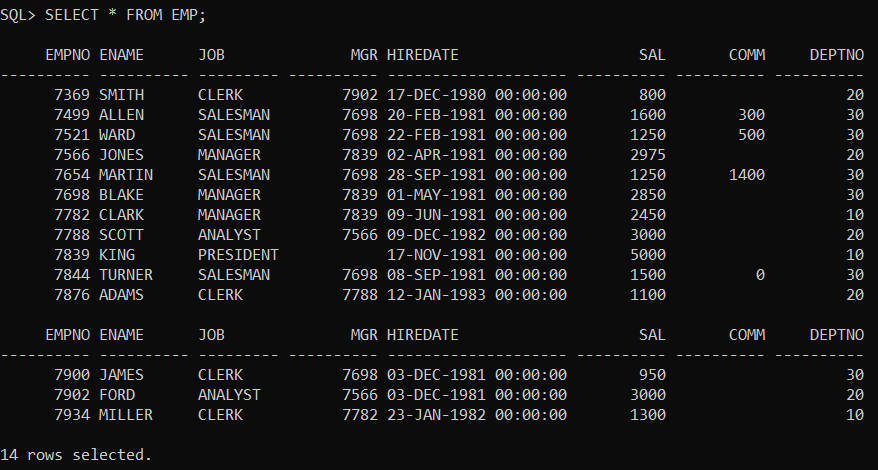Please use the below SQL script to create and populate the EMP table with the required data.

```CREATE TABLE EMP
(EMPNO NUMBER(4) NOT NULL,
ENAME VARCHAR2(10),
JOB VARCHAR2(9),
MGR NUMBER(4),
HIREDATE DATE,
SAL NUMBER(7, 2),
COMM NUMBER(7, 2),
DEPTNO NUMBER(2));

INSERT INTO EMP VALUES
(7369, 'SMITH', 'CLERK', 7902,
TO_DATE('17-DEC-1980', 'DD-MON-YYYY'),  800, NULL, 20);
INSERT INTO EMP VALUES
(7499, 'ALLEN',  'SALESMAN',  7698,
TO_DATE('20-FEB-1981', 'DD-MON-YYYY'), 1600,  300, 30);
INSERT INTO EMP VALUES
(7521, 'WARD',   'SALESMAN',  7698,
TO_DATE('22-FEB-1981', 'DD-MON-YYYY'), 1250,  500, 30);
INSERT INTO EMP VALUES
(7566, 'JONES',  'MANAGER',   7839,
TO_DATE('2-APR-1981', 'DD-MON-YYYY'),  2975, NULL, 20);
INSERT INTO EMP VALUES
(7654, 'MARTIN', 'SALESMAN',  7698,
TO_DATE('28-SEP-1981', 'DD-MON-YYYY'), 1250, 1400, 30);
INSERT INTO EMP VALUES
(7698, 'BLAKE',  'MANAGER',   7839,
TO_DATE('1-MAY-1981', 'DD-MON-YYYY'),  2850, NULL, 30);
INSERT INTO EMP VALUES
(7782, 'CLARK',  'MANAGER',   7839,
TO_DATE('9-JUN-1981', 'DD-MON-YYYY'),  2450, NULL, 10);
INSERT INTO EMP VALUES
(7788, 'SCOTT',  'ANALYST',   7566,
TO_DATE('09-DEC-1982', 'DD-MON-YYYY'), 3000, NULL, 20);
INSERT INTO EMP VALUES
(7839, 'KING',   'PRESIDENT', NULL,
TO_DATE('17-NOV-1981', 'DD-MON-YYYY'), 5000, NULL, 10);
INSERT INTO EMP VALUES
(7844, 'TURNER', 'SALESMAN',  7698,
TO_DATE('8-SEP-1981', 'DD-MON-YYYY'),  1500,    0, 30);
INSERT INTO EMP VALUES
TO_DATE('12-JAN-1983', 'DD-MON-YYYY'), 1100, NULL, 20);
INSERT INTO EMP VALUES
(7900, 'JAMES',  'CLERK',     7698,
TO_DATE('3-DEC-1981', 'DD-MON-YYYY'),   950, NULL, 30);
INSERT INTO EMP VALUES
(7902, 'FORD',   'ANALYST',   7566,
TO_DATE('3-DEC-1981', 'DD-MON-YYYY'),  3000, NULL, 20);
INSERT INTO EMP VALUES
(7934, 'MILLER', 'CLERK',     7782,
TO_DATE('23-JAN-1982', 'DD-MON-YYYY'), 1300, NULL, 10);
```
##### Oracle SUM Function:

The SUM Aggregate Function in Oracle is used to return the total sum of a given numeric column. The SUM function will only work on numeric data types. For columns containing string values, it will always return 0. Following is the syntax to use the SUM Function in Oracle.

Syntax: SELECT SUM(column) FROM TableName;

Example:
SELECT SUM(SAL) FROM EMP;
Output: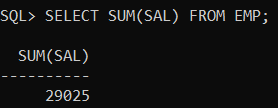Example:
SELECT SUM(SAL) FROM EMP WHERE JOB=’CLERK’;
Output: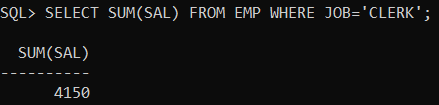##### AVG() Function in Oracle:

The AVG function in Oracle is used to return the average value of the given numeric column. The AVG function will only work on numeric columns. For columns containing string values, it will always return 0. Following is the syntax to use the AVG function in oracle.

Syntax: SELECT AVG(columnname) FROM tablename;

Example:
SELECT AVG(SAL) FROM EMP;
Output: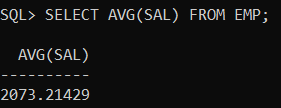Example:
SELECT AVG(SAL) FROM EMP WHERE DEPTNO=10;
Output: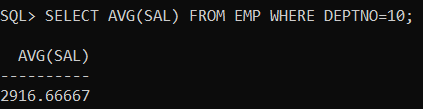##### MIN() Function in Oracle:

The MIN function in Oracle is used to return the smallest value of the given column. The MIN function works on numeric columns as well as string columns. The following is the syntax to use of MIN function in Oracle.

Syntax: SELECT MIN(columnname) FROM tablename;

Example:
SELECT MIN(HIREDATE) FROM EMP;
Output: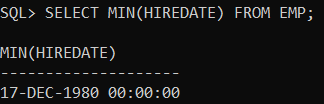Example:
SELECT MIN(HIREDATE) FROM EMP WHERE JOB=’MANAGER’;
Output: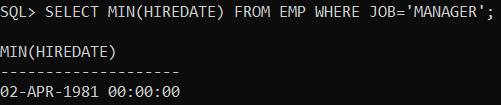Example:
SELECT MIN(SAL) FROM EMP;
Output:##### MAX() Function in Oracle:

The MAX function in Oracle is used to return the largest value of the given column. The MAX function works on numeric columns as well as string columns. The following SQL syntax shows how to use of MAX function in Oracle.

Syntax: SELECT MAX(columnname) FROM tablename;

Example:
SELECT MAX(HIREDATE) FROM EMP;
Output: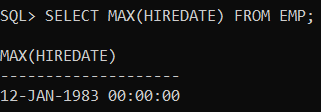Example:
SELECT MAX(HIREDATE) FROM EMP WHERE JOB=’MANAGER’;
Output: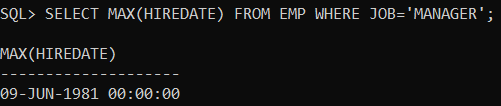Example:
SELECT MAX(SAL) FROM EMP;
Output: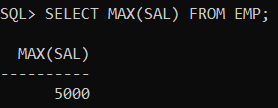##### COUNT() Function in Oracle:

The COUNT function in Oracle is used to count the data rows returned in the result set. The Oracle COUNT function counts distinct or all values in data rows returned in a result set. We can use the COUNT function in three ways as follows.

COUNT(*)
COUNT(<COLUMN NAME>)
COUNT(DISTINCT <COLUMN NAME>)

###### Example: Count the Total Number of employees in the Emp table.

Now, let’s count the data rows in the EMP table using the COUNT function in Oracle by executing the below SQL Statement.
SELECT COUNT(*) FROM EMP;
Output: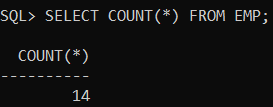In the next article, I am going to discuss the COUNT Function in Oracle with Examples. Here, in this article, I try to explain Multiple Row Functions in Oracle with Examples and I hope you enjoy this article.